# What are the rules for adding and subtracting negative numbers?

• Last Updated : 22 Dec, 2021

Algebra can be defined as the branch of mathematics which deals with the study, alteration, and analysis of various mathematical symbols. It is the study of unknown quantities, which are often depicted with the help of variables in mathematics. Algebra has a plethora of formulas and identities for the purpose of studying situations involving variables. It also has various sub-branches such as linear algebra, advanced algebra, commutative algebra, etc.

Numbers

Numbers are defined as quantities on which various mathematical operators, such as addition, subtraction, multiplication, and division can be applied. Not only are numbers used in mathematical practice but they also play a crucial role in our daily lives. The fields of accounting, economics, finance, stock markets, marketing, etc. also use numbers as their primary tool for analysis and interpretation.

Negative Numbers

In mathematics, such numbers as fall towards the left side of the number zero on the real number line are called negative numbers. Their position towards the left of zero indicates that their value is less than that of zero and hence they are written with the minus (−) sign before them.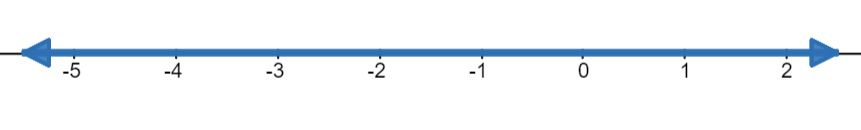The above picture depicts a number line showing some positive and negative integers. The numbers to the right of zero, i.e., positive numbers go on increasing in value from left to right.  Whereas the numbers to the left of zero (negative numbers) go on diminishing in value from right to left or increasing in value from left to right. Hence, −1 > −2. Hence a general rule can be formed here:

−(a) > −(a + 1)

### What is the rule for adding and subtracting negative numbers?

Solution:

For beginners, it is convenient to use a number line when performing addition and subtraction on negative numbers. To add and subtract, start by counting from zero on the number line. If the number from which the other number is being subtracted is negative, then start adding from zero towards left until the said number is obtained. Now, from that number start counting further towards left until the number which is to be subtracted is obtained.

Example: Solve: −1 + (− 2).

Step 1. Count one place towards left from zero.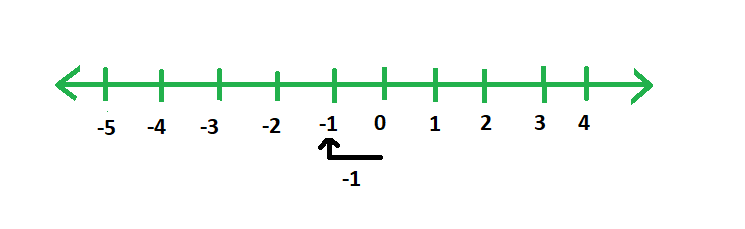Step 2. Count two places further towards the left from −1.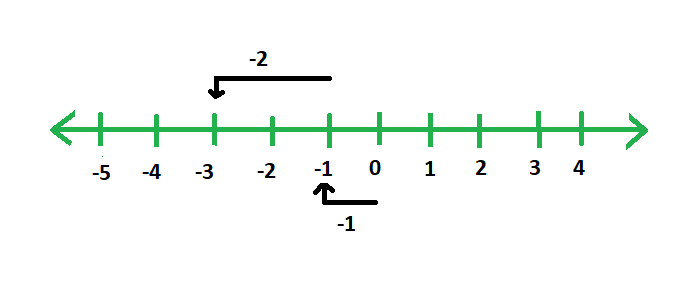This shows that: −1 + (− 2) = −3.

### Similar Problems

Question 1. Solve −1 (2).

Solution:

−1 − (−2) = −1 + 2

Step 1. Count one place towards left from zero.Step 2. Count two places towards the right from −1.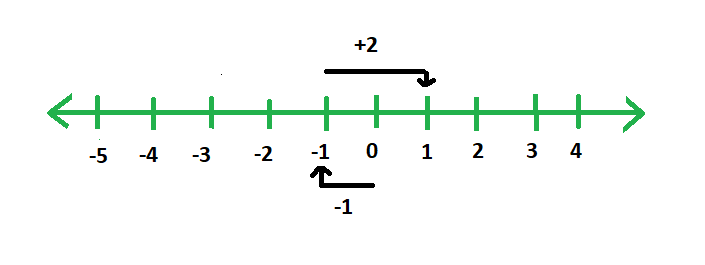This shows that: −1 − (−2) = 1.

Question 2. Solve −2 − (−3).

Solution:

−2 − (−3) = −2 +3

Step 1. Count two places towards left from zero.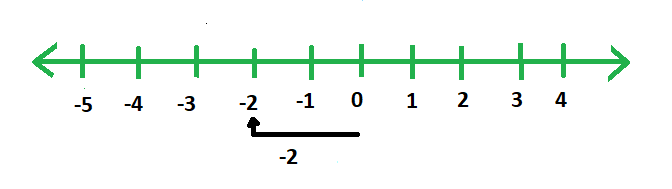Step 2. Count three places towards the right from −2.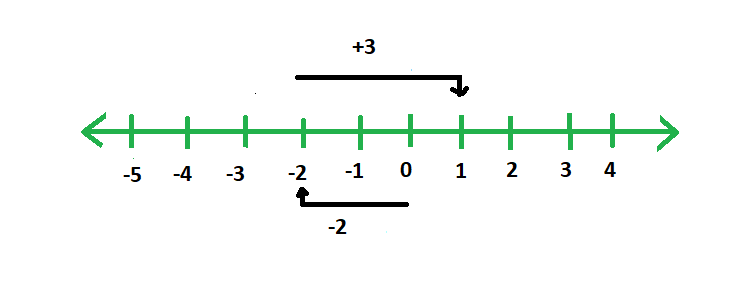This shows that: −2 +3 = 1.

Question 3. Solve −1 + (−4).

Solution:

Step 1. Count one place towards left from zero.Step 2. Count 4 places towards the left from −1.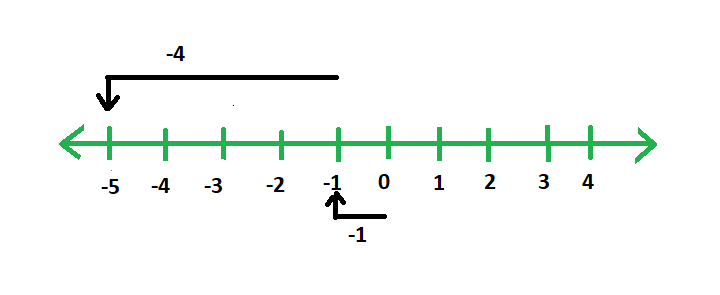This shows that: −1 + (−4) = −5.

Question 4. Solve −1 + (−3).

Solution:

Step 1. Count one place towards left from zero.Step 2. Count 3 places towards the left from −1.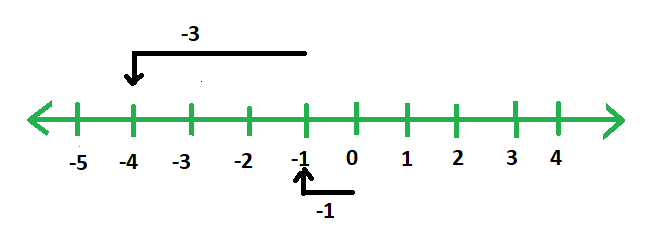This shows that: −1 + (−3) = −4.

Question 5. Solve −2 + 4.

Solution:

Step 1. Count two places towards left from zero.Step 2. Count 4 places towards the right from −2.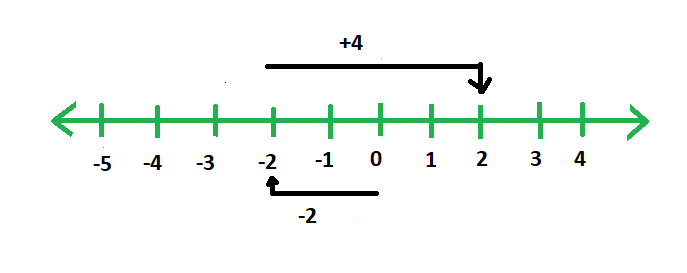This shows that −2 + 4 = 2.

My Personal Notes arrow_drop_up